# Time + fundamentals of Physics - math problems

#### Number of problems found: 89

• Cooker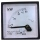A current of 2A passes through the immersion cooker at a voltage of 230V. What work do the electric field forces in 2 minutes?
• The shooter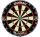The shooter heard the impact of the bullet on the target in one second after the shot. The bullet was moving at an average speed of 500 m/s. Calculate with speed of sound of 340 m/s. Determine the distance of the target.
• Electric workCalculate the work done by the electric forces passing the current of 0.2 A through the bulb in 10 minutes if the bulb is connected to a 230 V power supply.
• Motorcycle engineThe motorcycle engine has a constant power of 1.2 kW for 0.5 hours. How much work does the engine do? Express work in kWh and Ws units.
• A particle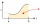A particle moves in a straight line so that its velocity (m/s) at time t seconds is given by v(t) = 3t2-4t-4, t>0. Initially the particle is 8 meters to the right of a fixed origin. After how many seconds is the particle at the origin?
• Self-oscillation period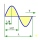The water in the vessel carried by the boy has a self-oscillation period of 0.8 s. What is the size of the boy's movement speed when the length of the boy's step is 60 cm? Give the result in m/s.
• The tourist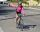The tourist came started from the hostel at an average speed of 5km/h. Half an hour later, the bicyclist started along the same route at a speed of 20km/h. How many minutes will a cyclist catch up and how many kilometers will he go?
• Two trains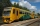From station A, the freight train traveled at a speed of 40 km/h in 9h. When he drove 15km, the fast train started from station A in the same direction at a speed of 70km/h. When will it the freight train catch up?
• Candles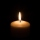Before Christmas, Eva bought two cylindrical candles - red and green. Red was 1 cm longer than green. She lit a red candle on Christmas Day at 5:30 p. M. , lit a green candle at 7:00 p. M. , and left them both on fire until they burned. At 9:30 p. M. , bo
• GravitationFrom the top of the 80m high tower, the body is thrown horizontally with an initial speed of 15 m/s. At what time and at what distance from the foot of the tower does the body hit the horizontal surface of the Earth? (use g = 10 ms-2)
• The coal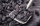The coal stock would be enough to heat a larger room for 12 weeks, a smaller one for 18 weeks. It was heated for four weeks in both rooms, then only in a smaller one. How long was the coal stock enough?
• A carA car weighing 1.05 tonnes driving at the maximum allowed speed in the village (50 km/h) hit a solid concrete bulkhead. Calculate height it would have to fall on the concrete surface to make the impact intensity the same as in the first case!
• Two citiesThe car goes from city A to city B at an average speed of 70 km/h, back at an average speed of 50 km/h. If it goes to B and back at an average speed of 60 km/h, the whole ride would take 8 minutes less. What is the distance between cities A and B?
• Friction coefficientWhat is the weight of a car when it moves on a horizontal road at a speed of v = 50 km/h at engine power P = 7 kW? The friction coefficient is 0.07
• Two airportsTwo airports are 2690 km away. From the first airport, the airplane flies at a speed of 600 km/h, from a second airplane at a speed of 780 km/h. When will they meet if they flew at 10:00? How far from the first airport?
• Train delay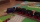Due to a breakdown, the train lost 16 minutes of standing on the track behind Brno. He "eliminated" this delay so that after the start, the 80 km long section went at a speed 10 km/h higher than originally planned. What speed was it and what was it suppos
• Two aircraftFrom the airport will start simultaneously two planes, which fly tracks are perpendicular to each other. The first flying speed of 680 km/h and the second 840 km/h. Calculate how far the aircraft will fly for half an hour.
• Athletic competitionIn a 400 meter athletic competition, a participant covers the distance as given below. find the average speed? first 80 meters 10 m/s next 240 meters 7.5 m/s last 80 meters 10 m/s
• Rocket start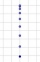The body launched vertically up returns to the start site in 6 seconds. What height did it have?
• Orlík hydroelectric plantThe Orlík hydroelectric power plant, built in 1954-1961, consists of four Kaplan turbines. For each of them, the water with a flow rate of Q = 150 m3/s is supplied with a flow rate of h = 70.5 m at full power. a) What is the total installed power of the p

Do you have an interesting mathematical word problem that you can't solve it? Submit a math problem, and we can try to solve it.

We will send a solution to your e-mail address. Solved examples are also published here. Please enter the e-mail correctly and check whether you don't have a full mailbox.

Please do not submit problems from current active competitions such as Mathematical Olympiad, correspondence seminars etc...

Do you want to convert time units like minutes to seconds?